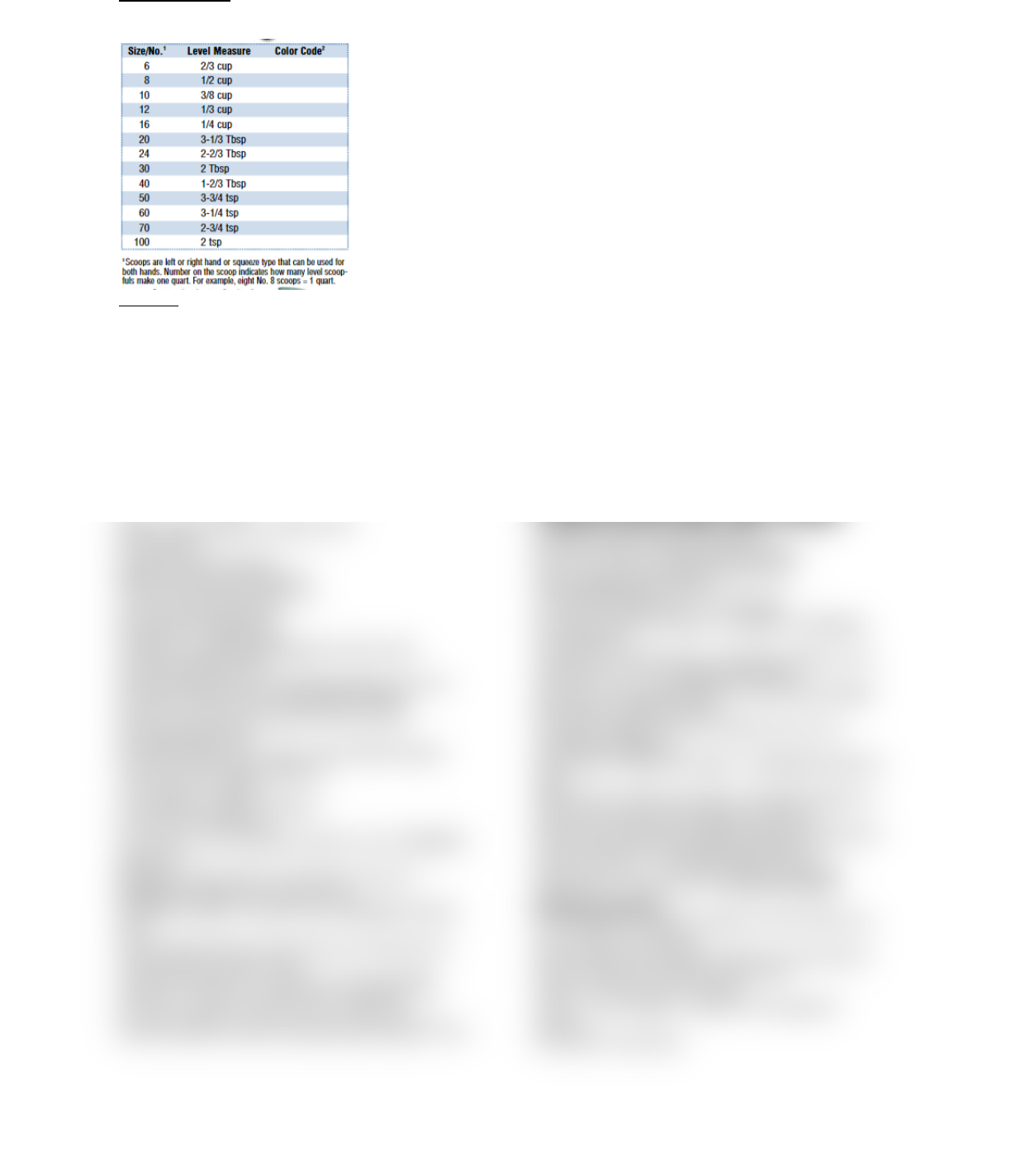Study Guides (400,000)
US (230,000)
PSU (3,000)
HM (8)
HM 329 (1)
Final

# HM 329 Study Guide - Final Guide: Pastry Chef, Maillard Reaction, Caramelization

Department
Hospitality Management
Course Code
HM 329
Professor
Professor Kim
Study Guide
Final

This preview shows half of the first page. to view the full 2 pages of the document.Unit conversions:
1 gallon = 4 quarts = 8 pints = 16 cups=8 ounces
1lb.(1#)=16 ounces
Formulas
Variable Cost
=prior period total cost/prior period # meals
=prior period cost per meal+estimated increase
=budgeted cost per meal x projected # meals
Contribution margin
=total sales-variable costs
Contribution margin %
=contribution margin \$/total sales
=contribution margin per unit/selling price per unit
Break even in units
=fixed costs/contribution margin in dollars per meal
Break even in dollars
=fixed costs/contribution margin percent
Pre tax profit
=after tax profit/(1-tax rate)
Break even plus desired profit
=(fixed costs+desired profit)/cm %
Revenue-Expenses=Profit
Expense/Rev = Expense %
Profit/Rev = Profit % (anything/rev= %) Rev-Ideal
Expense=Desired Profit
total sales/# guests served=Check Average guest count
forecast x forecast check average=Rev Forecast
total # of menu item sold/ total # of all menu items
sold=Popularity Index
forecasted # guest x pop. Index = prod. Amnt forecast
Product Loss/AP weight = Waste %
1.00- waste % = Yield %
EP quantity/AP quantity = Yield %
AP cost/ Yield %=EP cost
Prior period’s cost +Estimated increase in cost = Budgeted
fixed cost
Budgeted cost per meal x # of meals to be served =
Budgeted variable Prior period’s cpm
Estimated increase in cost per meal = Budgeted cost per
meal
Prior period’s total cost / Prior period’s # of meals served
=Prior period’s cost per meal
Usage during lead time + safety stock= Par Stock Min
Par value on hand + special order = Order amt
Inventory Valuation: Item Amnt x Item Value (LIFO 1st
items purchased recorded as sold(not good indicator), FIFO-
last items purchased recorded as sold) = Item Inventory
Value
Beginning Inventory + Purchases = Goods avail for sale
Goods avail for sale ending inventory = Cost of Food
Consumed
Cost of Food Consumed Employee Meals = Cost of Food
Sold
Cost of Food Sold/ Food sales = Food Cost % # of items
sold x standard cost = Attainable Cost
Actual Product Cost /Attainable Product Cost = Operational
Effic. Ratio
(actual food cost attainable food cost/attainable food cost =
cost% variance
Product Cost EP/AP amt x EP/AP Cost = Ingred. Cost
Total Ingred. Costs = Recipe Standard Cost
Recipe Total Cost/# of Portions= Portion Cost
Portion Cost/Menu Price =Item Food Cost %
1.00/(Desired) Product Cost % =Mark-up Factor
Mark-up Factor x Product Cost = Min Menu Price
Portion Cost + Contrib. Margin = Min Selling Price
Selling Price Product Cost = Contribution Margin
Total Buffet Product Cost/Guests Served = Buffet Product
Cost per Guest
Buffet Product CPG/ Desired food cost % = Buffet Selling
price per Guest
Cost of labor: Output/Input = Productivity Ratio
Cost of Labor/Total sales= Labor Cost %
Total Sales/Labor hrs used=Sales per Labor Hr
step 1: Cost of Labor/Guests Serves = Labor \$ per Guest
Served
3 Guests Served/Cost of Labor or Labor Hours used =
Guests served per Labor \$orLabor hr
Rev/Avail. Seat hrs = Rev per Avail. Seat Hr
step 2: Cost of labor Budget/forecasted total
sales=Budgeted labor cost %
4 actual amt/budgeted amt = % of Budget
# of Employ. Sep/# of Employ. In workforce = Employee
Turnover Rate
Total Sales - Variable Costs = Contribution Margin Av # of
Entrees Sold x 0.7 = Acceptable Popularity
Fixed Cost / Contribution Margin per Unit (Guest)= Break-
Even Point in Guests Served
Contribution Margin per Unit / Selling Price per Unit =
Contribution Margin %
Fixed Costs / Contribution Margin % =Break-Even Point in
Sales
(Fixed Costs + Before-Tax Profit) / Contribution Margin %=
Sales \$ to Achieve Desired Before-Tax Profit
(Fixed Costs + Before-Tax Profit)/Contribution Margin/Unit=
Covers to Achiev. Desired Before-Tax Profit
After Tax Profit / (1 Tax Rate)= Before Tax Profit
Selling Price Product Cost = Contribution Margin
Recipe Conversions
As Purchase (AP Quantity): quantity of an item before any
trim or waste are considered
Edible Portions (EP Quantity): quantity of an item after all
trim and waste has been taken into account
Waste %: Product Loss/ AP Weight
Yield %: 1.00 Waste % | Yield %= EP Quantity/AP
Quantity
EP Cost=AP Cost/Yield%
find more resources at oneclass.com
find more resources at oneclass.com